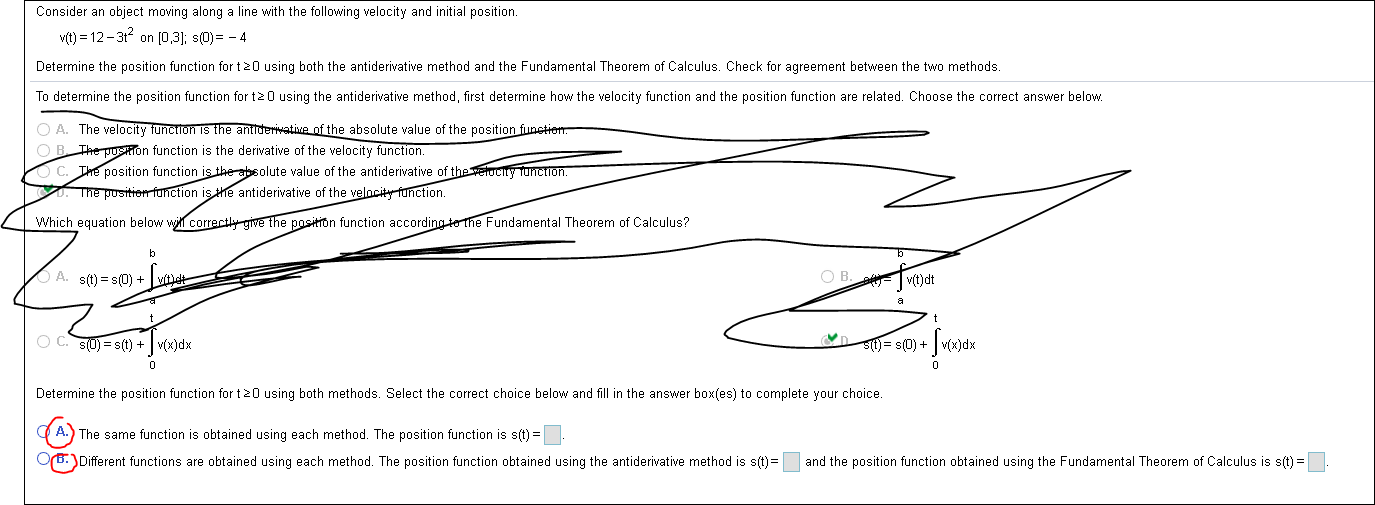# Consider an object moving along a line with the following velocity and initial position.vit)12-3t2 on [0,3]; s(D)-4Determine the position function for t 20 using both the antiderivative method and the Fundamental Theorem of Calculus. Check for agreement between the two methods.To determine the position function for t 0 using the antiderivative method, first determine how the velocity function and the position function are related. Choose the correct answer below.OA. The velocity function is the antiderivative of the absolute value of the position funetiom.OB The pusTon function is the derivative of the velocity function.C. The position function is the absolute value of the antiderivative of the verocity Tunction.The pusitien function is he antiderivative of the velncity function.Which equation below will correcthy give the position function according to the Fundamental Theorem of Calculus?O B. vit)dtA. s(t) s(0)+nO C. SUs(t) v(x)dxSttj= s(0)+Determine the position function for t20 using both methods. Select the correct choice below and fill in the answer box(es) to complete your choice.A. The same function is obtained using each method. The position function is s(t)OrBDifferent functions are obtained using each method. The position function obtained using the antiderivative method is s(t)and the position function obtained using the Fundamental Theorem of Calculus is s(t)

Question
327 views

I keep trying hahah.help_outlineImage TranscriptioncloseConsider an object moving along a line with the following velocity and initial position. vit)12-3t2 on [0,3]; s(D)-4 Determine the position function for t 20 using both the antiderivative method and the Fundamental Theorem of Calculus. Check for agreement between the two methods. To determine the position function for t 0 using the antiderivative method, first determine how the velocity function and the position function are related. Choose the correct answer below. OA. The velocity function is the antiderivative of the absolute value of the position funetiom. OB The pusTon function is the derivative of the velocity function. C. The position function is the absolute value of the antiderivative of the verocity Tunction. The pusitien function is he antiderivative of the velncity function. Which equation below will correcthy give the position function according to the Fundamental Theorem of Calculus? O B. vit)dt A. s(t) s(0) + n O C. SUs(t) v(x)dx Sttj= s(0)+ Determine the position function for t20 using both methods. Select the correct choice below and fill in the answer box(es) to complete your choice. A. The same function is obtained using each method. The position function is s(t) OrBDifferent functions are obtained using each method. The position function obtained using the antiderivative method is s(t) and the position function obtained using the Fundamental Theorem of Calculus is s(t) fullscreen
check_circle

Step 1

To determine the correct choice from (A) and (B) by calculating the position function using antiderivative method and fundamental theorem of calculus. Now, for the correct choice between (A) and (B) first calculate the position function by integrating the velocity function with taking the limit of t as [0, t] and use the initial condition s(0)=-4. The provided velocity function is v(t)=12-3t2.

Step 2

Now, for the antiderivative method integrate the provided velocity function v(t)=12-3t2.

Step 3

Thus, the position function by the antid...

### Want to see the full answer?

See Solution

#### Want to see this answer and more?

Solutions are written by subject experts who are available 24/7. Questions are typically answered within 1 hour.*

See Solution
*Response times may vary by subject and question.
Tagged in

### Integration